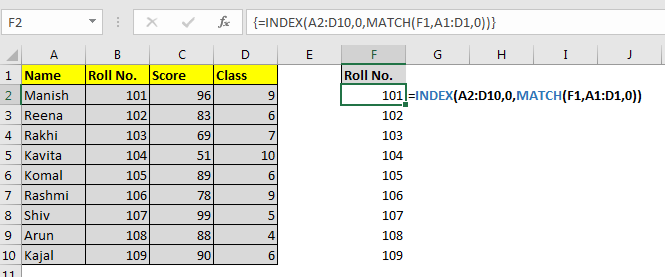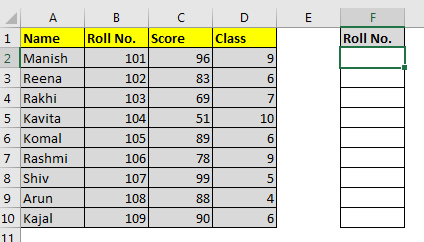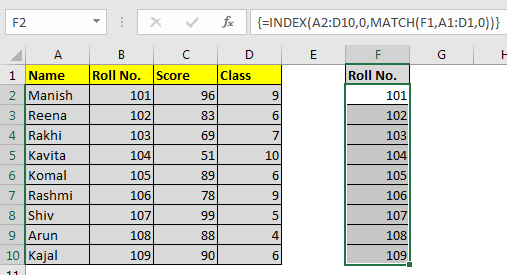# How to Lookup Entire Column in Excel Using Index Match

Sometimes we want to lookup entire columns using some key or lookup value in Excel. In that case you may use traditional HLOOKUP formula or INDEX MATCH formula multiple times. But we can retrieve entire column in one hit using INDEX-MATCH. Let’s see how.Generic Formula to Lookup Entire Row

{=INDEX(table,0,MATCH(lookup value, lookup range),0)}

Note: this a multicell array formula and must be entered with CTRL+SHIFT+ENTER while having output range selected.

Table: the reference of entire table that contains the columns you want to retrieve.
Lookup value: the key for lookup.
Lookup range: the row that contains the lookup value. Mostly the headings.

Let’s see an example to make things clear.
Example: Retrieve All Roll Numbers From Students Table
Here I have some students data in excel sheet. I want to retrieve entire roll number column using heading name Roll No.We want the output in range F2:F10. Select the range F2:F10 and write this formula.

{=INDEX(A2:D10,0,MATCH(F1,A1:D1,0))}

Hit CTRL+SHIFT+ENTER. This makes this formula a multicell array formula.
You will see the entire column retrieved.How it works?

Well its a basic INDEX-MATCH function. The only difference is that we have given reference of entire table to index instead of one row only. Let’s break it down to understand.

MATCH(F1,A1:D1,0): The match looks for the value of F1 (Roll No.) in range A1:D1. It is found at 2nd index. Hence it returns 2. So now the formula is =INDEX(A2:D10,0,2)
INDEX(A2:D10,0,2): The INDEX function looks at 2nd Column in range A2:D10 and returns the entire column value in an array.{101;102;103;104;105;106;107;108;109}.
When we enter it as an single cell array formula it only returns the first value of array. But when we select multiple cells, each value occupies a cell to show case itself.

So yeah guys, this how you can retrieve entire Column in excel using INDEX MATCH formula. Let me know if you have any doubts regarding this excel formula or any other feature of excel or vba. Right down in the comments section below.

Related Articles:

How to Lookup Entire Row Using Index Match

How to retrieve the entire row of a matched value

How to Select Entire Column and Row Using Keyboard Shortcuts in Excel

Sum across entire column

Popular Articles:

The VLOOKUP Function in Excel

COUNTIF in Excel 2016

How to Use SUMIF Function in Excel

Terms and Conditions of use

The applications/code on this site are distributed as is and without warranties or liability. In no event shall the owner of the copyrights, or the authors of the applications/code be liable for any loss of profit, any problems or any damage resulting from the use or evaluation of the applications/code.